# Pontryagin maximum principle

(diff) ← Older revision | Latest revision (diff) | Newer revision → (diff)

Relations describing necessary conditions for a strong maximum in a non-classical variational problem in the mathematical theory of optimal control (cf. Optimal control, mathematical theory of). It was first formulated in 1956 by L.S. Pontryagin (see ).

The proposed formulation of the Pontryagin maximum principle corresponds to the following problem of optimal control. Given a system of ordinary differential equations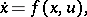(1)

whereis a phase vector,is a control parameter andis a continuous vector function in the variablesthat is continuously differentiable with respect to. A certain setof admissible values of the control parameterin the space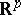is given; two points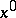and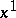in the phase spaceare given; the initial timeis fixed. Any piecewise-continuous function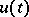,, with values in, is called an admissible control. One says that an admissible control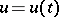transfers the phase point from the positionto the position(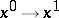) if the corresponding solution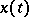of the system (1) satisfying the initial condition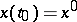is defined for all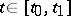and if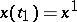. Among all admissible controls transferring the phase point from the positionto the positionit is required to find an optimal control, i.e. a function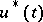for which the functional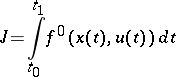(2)

takes least possible value. Here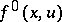is a given function from the same class as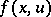,is the solution of the system (1) with the initial conditioncorresponding to the control, andis the time at which this solution passes through. The problem consists of finding a pair consisting of the optimal controland the corresponding optimal trajectory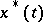of (1).

Let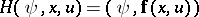be a scalar function (Hamiltonian) of the variables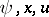, where,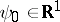,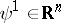,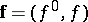. To the function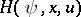corresponds a canonical (Hamiltonian) system (with respect to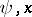)(3)

(the first equation in (3) is the system (1)). Let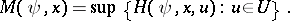The Pontryagin maximum principle states: If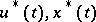() is a solution of the optimal control problem (1), (2) (,), then there exists a non-zero absolutely-continuous function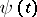such thatsatisfy system (3) in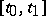, such that for almost-allthe function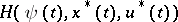attains its maximum: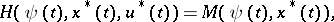(4)

and such that at the terminal timethe conditions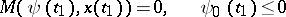(5)

are satisfied.

If the functionssatisfy the relations (3), (4) (i.e.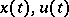are Pontryagin extremal), then the conditions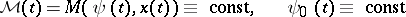hold.

From the above statement follows the maximum principle for the time-optimal problem (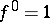,). This statement admits a natural generalization to non-autonomous systems, problems with variable end-points and problems with restricted phase coordinates (, whereis a closed set in the phase spacesatisfying some additional restrictions (see )).

Admitting closed sets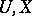(in particular, these regions can be determined by systems of non-strict inequalities) makes the problem under consideration non-classical. The fundamental necessary conditions from the classical calculus of variations with ordinary derivative follow from the Pontryagin maximum principle (see  and also Weierstrass conditions (for a variational extremum)).

A widely used proof of the above formulation of the Pontryagin maximum principle, based on needle variations (i.e. one considers admissible controls arbitrarily deviating from the optimal one but only on a finite number of small time intervals), consists of linearization of the problem in a neighbourhood of the optimal solution, construction of a convex cone of variations of the optimal trajectory, and subsequent application of the theorem on separated convex cones (see ). The corresponding condition is then rewritten in the analytical form (3), (4) in terms of the maximum of the Hamiltonianof the phase variables, the controlsand the adjoint variables, which play the same role as the Lagrange multipliers in the classical calculus of variations. Effective application of the Pontryagin maximum principle often necessitates the solution of a two-point boundary value problem for (3).

The most complete solution of the problem of optimal control was obtained in the case of certain linear systems, for which the relations in the Pontryagin maximum principle are not only necessary but also sufficient optimality conditions.

There are numerous generalizations of the Pontryagin maximum principle; for instance, in the direction of more complicated non-classical constraints (including mixed constraints imposed on the controls and phase coordinates, functional and different integral constraints), in studies of the sufficiency of the corresponding constraints, in the consideration of generalized solutions, so-called sliding regimes, systems of differential equations with non-smooth right-hand side, differential inclusions, optimal control problems for discrete systems and systems with an infinite number of degrees of freedom, in particular, described by partial differential equations, equations with an after effect (including equations with a delay), evolution equations in a Banach space, etc. The latter lead to new classes of variations of the corresponding functionals, the introduction of the so-called integral maximum principle, the linearized maximum principle, etc. Rather general classes of variational problems with non-classical constraints (including non-strict inequalities) or with non-smooth functionals are usually called problems of Pontryagin type. The discovery of the Pontryagin maximum principle initiated the development of mathematical optimal control theory. It stimulated new research in the field of differential equations, functional analysis and extremal problems, computational mathematics and other related domains.

How to Cite This Entry:
Pontryagin maximum principle. Encyclopedia of Mathematics. URL: http://encyclopediaofmath.org/index.php?title=Pontryagin_maximum_principle&oldid=13246
This article was adapted from an original article by A.B. Kurzhanskii (originator), which appeared in Encyclopedia of Mathematics - ISBN 1402006098. See original article